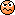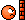# Kinematics Projectile Motion Problem

• joeyoung1996
In summary, the ball was launched from ground level and landed 2.85 seconds later on a level field 48 meters away. The ball had an initial velocity of 16.84 meters per second and an angle above the horizontal of 16.22 degrees.f

## Homework Statement

A ball launched from ground level lands 2.85s later on a level field 48 m away from the launch point. Find the magnitude of the initial velocity vector and the angle it is above the horizontal. (Ignore any effects due to air resistance.)

## Homework Equations

##x=x_0+v_{0x}t+\frac{1}{2}at^2##
##y=y_0+v_{0y}t+\frac{1}{2}at^2##

## The Attempt at a Solution

So, I worked out this problem seemingly without an issue, but WebAssign keeps telling me the answers are wrong, so maybe a bit of new eyes can point out what I've done wrong.

I start by attempting to solve for the x component of the velocity vector:
##x=x_0+v_{0x}t+\frac{1}{2}at^2##
##48m=0m+v_{0x}(2.85s)+\frac{1}{2}(0)(2.85s)^2##
##48m=v_{0x}(2.85s)##
##v_{0x}=\frac{48m}{2.85s}##
##v_{0x}=16.84\frac{m}{s}##
I then found the y component of the velocity:

##y=y_0+v_{0y}t+\frac{1}{2}at^2##
##0m=0m+v_{0y}(2.85s)+\frac{1}{2}(-9.8\frac{m}{s^2})(2.85s)^2##
##0=v_{0y}(2.85s)-13.96m##
##v_{0y}=\frac{13.96m}{2.85s}##
##v_{0y}=4.9\frac{m}{s}##
Now that I have both components, I use the Pythagorean Theorem to find the magnitude of the velocity:

##||\vec{v_0}|| = \sqrt{v_{0x}^2+v_{0y}^2}##
##||\vec{v_0}||= \sqrt{(16.84\frac{m}{s})^2+(4.9\frac{m}{s})^2}##
##||\vec{v_0}||=17.5\frac{m}{s}##
Finally, I use these to find the angle at which the ball was fired:

##sinx=\frac{4.9}{17.5}##
##x=sin^{-1}(\frac{4.9\frac{m}{s}}{17.5\frac{m}{s}})##
##x=16.22^{\circ}##
I figured I have just made a simple, stupid mistake, but for the life of me, I cannot find it.Last edited:
I then found the y component of the velocity:

##y=y_0+v_{0y}t+\frac{1}{2}at^2##
##0m=0m+v_{0y}(2.85s)+\frac{1}{2}(-9.8\frac{m}{s^2}(2.85s)^2##

Did you forget to square the time in the last term above? Otherwise, your work looks good to me.

LOL I didn't square the time haha! I feel silly now; thank you so much!

You're welcome. It was just a careless error, like we all make. Hope it works out now.